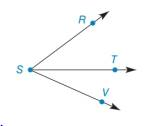Chapter 1.4, Problem 21E### Elementary Geometry for College St...

6th Edition
Daniel C. Alexander + 1 other
ISBN: 9781285195698

#### Solutions

Chapter
Section### Elementary Geometry for College St...

6th Edition
Daniel C. Alexander + 1 other
ISBN: 9781285195698
Textbook Problem
1 views

#Exercises 16 − 24 Given: m ∠ R S T = x 2 m ∠ T S V = x 4 m ∠ R S V = 45 ° Find: x     and m ∠ R S T

To determine

To find:

The value of x and the measure of the angle RST.

Explanation

Given:

mRST=x2mTSV=x4mRSV=45°

The given figure is,

Definition:

If a point say M lies in the interior of PQR, then mPQM+mRQM=mPQR.

Calculation:

Consider the figure,

T is the interior point of the angle RSV.

mRST=x2mTSV=x4mRSV=45°

Use above mentioned angle addition postulate to solve for the value of x.

### Still sussing out bartleby?

Check out a sample textbook solution.

See a sample solution

#### The Solution to Your Study Problems

Bartleby provides explanations to thousands of textbook problems written by our experts, many with advanced degrees!

Get Started

#### Differentiate the function. f(x ) = sin(ln x)

Single Variable Calculus: Early Transcendentals, Volume I

#### 14. Write in logarithmic form.

Mathematical Applications for the Management, Life, and Social Sciences

#### True or False: is a convergent series.

Study Guide for Stewart's Multivariable Calculus, 8th

#### Explain the distinction between science and pseudoscience.

Research Methods for the Behavioral Sciences (MindTap Course List)